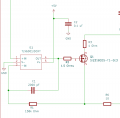# How to find op-amp gain

#### ahammerle

Joined Mar 20, 2023
14
I'm testing this current sink circuit (as shown below) and noticed the gain is railing against the +5V input. I need 300mA to sink across R3 and R6 at 3V. The filter is at 503Hz. The max input of the op-amp is 3.165V. That being said, I set the R6 to 10 ohms becuase (R_set = V_in/I_set). I only reviewed basic op-amps in class so I'm confused if R3 and R6 factor into the overall gain op-amp. If anyone can help that would be appreciated. I found this circuit here: Programmable low-side current sink circuit (ti.com)#### Ian0

Joined Aug 7, 2020
8,400
Can we have the rest of the circuit, please? It disappears off the right hand side.

#### crutschow

Joined Mar 14, 2008
32,913
You may not have enough voltage at the MOSFET gate (Vgs) to turn it sufficiently on.
What is the Vgs(th) value in its data sheet?

What is the measured voltage at its source terminal?

#### ahammerle

Joined Mar 20, 2023
14
You may not have enough voltage at the MOSFET gate (Vgs) to turn it sufficiently on.
What is the Vgs(th) value in its data sheet?

What is the measured voltage at its source terminal?
The Vgs(th) max is 2.5V and the measured voltage at source terminal is 2.93V.

#### WBahn

Joined Mar 31, 2012
29,165
If the non-inverting input of the op-amp is 3.165 V, the opamp is going to try to output a voltage to the gate of the NFET that is high enough for it to conduct enough current so as to get 3.165 V at the inverting input. But no matter how hard you turn on that FET, the absolute most you could possibly get at the source is 3 V (and you are getting close to that at 2.93 V). So I would expect the opamp to rail it's output since that's the closest it can come.

#### ahammerle

Joined Mar 20, 2023
14
If the non-inverting input of the op-amp is 3.165 V, the opamp is going to try to output a voltage to the gate of the NFET that is high enough for it to conduct enough current so as to get 3.165 V at the inverting input. But no matter how hard you turn on that FET, the absolute most you could possibly get at the source is 3 V (and you are getting close to that at 2.93 V). So I would expect the opamp to rail it's output since that's the closest it can come.
Hmm... I see that makes sense. I'm a bit confused because at the drain the voltage reads 2V for this circuit setup which means I'm only getting 200mA to sink. I wonder if modifying R6 to a higher value would help this problem?

#### crutschow

Joined Mar 14, 2008
32,913
at the drain the voltage reads 2V for this circuit setup which means I'm only getting 200mA
I'm confused.
2V at the drain means there is 1V across R3 which is 1A(?).
I wonder if modifying R6 to a higher value would help this problem?
No, that makes it worse, since it would reduce the maximum available Vgs.
You need to go in the the other direction and reduce the value of R6.

For example, since the maximum op amp output is 5V, and the MOSFET can require a Vgs of at least 2.5V, then the drop across R6 must be less than 2.5V.
So if you change R6 to 5Ω, its drop will be 1.5V at 300mA, giving a max Vgs of 5V-1.5V = 3.5V, which should be more than enough to supply the 300ma through the MOSFET.

This doubles the gain, but you can add a 2:1 resistive attenuator (two equal value resistors) at the input to give the same sensitivity, if you want.

Last edited:

#### WBahn

Joined Mar 31, 2012
29,165
Hmm... I see that makes sense. I'm a bit confused because at the drain the voltage reads 2V for this circuit setup which means I'm only getting 200mA to sink. I wonder if modifying R6 to a higher value would help this problem?
Your numbers aren't consistent. You said that the source voltage was 2.93 V. How can the drain voltage be 2 V in the same setup?

Earlier, you said that you used R_set = V_in/I_set, but you didn't specify which resistor, voltage, and current any of these three parameters refer to.

If V_in is your +3 V indicated in your second diagram, then it is NOT the voltage across ANY of the resistors. Ohm's Law is very specific -- it relates the current through a particular resistor and the voltage across THAT resistor to the resistance of THAT resistor.

If you want (as you stated in your original post) 300 mA to flow through (current flows through, not across, components) R3 and R6 when 3 V is applied to the node that you show it at, then your resistors are going to have to be smaller. As it is, with R3 = 1 Ω and R6 = 10 Ω, just those two resistors need a combined 3.1 V across them -- and then you need to have the Vds of the MOSFET,

Take a step back and describe what the problem is that you are trying to solve with an electronic circuit. Where is this 3.165 V value coming from? Where is this 3 V value coming from? What is the relationship that you are trying to achieve?